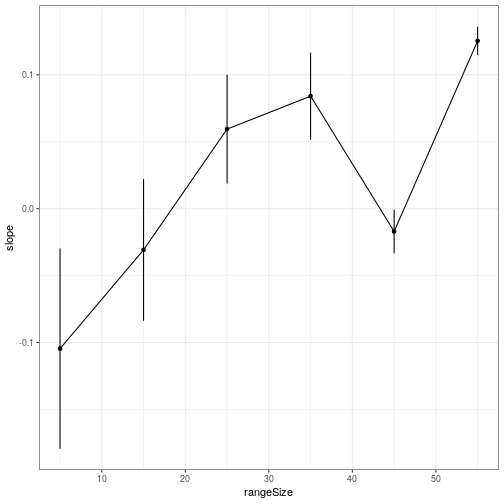## Appendix S5

Supporting information in Valcu, M., Dale, J., and Kempenaers, B. (2012). rangeMapper: a platform for the study of macroecology of life-history traits. Global Ecology and Biogeography 21, 945-951.

The example shown here is run on the wrens dataset which is part of the package. The wrens dataset has 84 species while the case study presented in the paper was run on 8434 bird species. Therefore both the settings and the results shown below are not identical with the results presented in Valcu et al 2012.

### Project Set Up

``````require(rangeMapper)
"extdata", "wrens", "vector_combined"), "wrens", verbose = FALSE)
data(wrens)
d = subset(wrens, select = c('sci_name', 'body_mass') )
con = ramp("wrens.sqlite", gridSize = 1, spdf = breding_ranges,
biotab = d, ID = "sci_name",metadata = rangeTraits()['Area'],
overwrite = TRUE)
``````
``````## New session 2019-10-25 17:52:42
``````
``````## PROJECT: wrens.sqlite
## DIRECTORY: /tmp/Rtmpe4D7pf
``````
``````## Grid size set to 1 map units.
``````
``````## Canvas uploaded.
``````
``````## Writing overlay output to project...
``````
``````## 84 out of 84 ranges updated; Elapsed time: 0 mins
``````
``````## Extracting metadata...
``````
``````## Table biotab saved as a  BIO_ table
``````
``````## +______________+
``````
``````## class            rangeMap
## Project_location /tmp/Rtmpe4D7pf/wrens.sqlite
## Proj4            +proj=longlat +ellps=GRS80 +towgs84=0,0,0,0,0,0,0 +no_defs
## CellSize         1
## Extent           xmin=-164.8716,xmax=-34.60225,ymin=-55.72056,ymax=61.5632
## BIO_tables       biotab
## MAP_tables       species_richness
``````
``````## +______________+
``````

Range size classes

``````mt = dbReadTable(con, "metadata_ranges")

Q = quantile(log(mt\$Area),  probs = seq(0.05, 1, 0.1) )
rangeA = data.frame(area = exp(Q), quant =  gsub("%", "", names(Q)) )
``````

### Run `log10(median_body_mass) ~ sqrt(species_richness)` regression for each range size interval

``````W = 4   # size of the moving window

output = vector(mode = "list", length = nrow(rangeA))
names(output) = rangeA\$quant

for(i in seq(1:(nrow(rangeA) - W) ) ) {

# Define  map subset
area_subset =list(metadata_ranges = paste("Area between",  rangeA[i,"area"],
"and", rangeA[i+W,"area"]))

# Save map
rangeMap.save(con, subset = area_subset , biotab = "biotab",
biotrait = "body_mass", FUN = "median", tableName = "median_body_mass",
overwrite = TRUE)

# Fetch map
m = rangeMap.fetch( con, c("species_richness", "median_body_mass"), spatial = FALSE )

# Perform OLS regression
# NOTE: In order to perform a spatial simultaneous autoregressive error
# regression
#  see 'errorsarlm ' function and the auxiliary neighbours list functions in
# 'spdep' package.
fm = lm( log10(median_body_mass) ~  sqrt(species_richness), m)

output[[i]] =  fm

}

output = output[!sapply(output, is.null)]
``````

### Extract Regression Parameters and plot

``````X = lapply(output, function(x) data.frame(slope = coef(x),
ciu = confint(x)[2,1], cil = confint(x)[2,2]) )
X = do.call(rbind, X)
X\$rangeSize = as.numeric(row.names(X))

# Plot

require(ggplot2)

ggplot(X, aes(x = rangeSize, y = slope)) +
geom_errorbar(aes(ymin = cil, ymax = ciu), width= 0) +
geom_line() +
geom_point() +
theme_bw()
``````